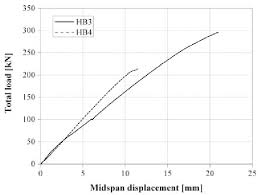## How to Calculate and Solve for Total Load on Composite | Composites

The total load on composite is illustrated by the image below.To compute for total load on composite, two essential parameters are needed and this parameters are Load on Matrix (Fm) and Load on Fibre (Ff).

The formula for calculating total load on composite:

Fc = Fm + Ff

Where:

Fc = Total Load on Composite

Let’s solve an example;
Find the total load on composite when the load on matrix is 20 and the load on fibre is 10.

This implies that;

Fm = Load on Matrix = 20
Ff = Load on Fibre = 10

Fc = Fm + Ff
Fc = (20) + (10)
Fc = 30

Therefore, the total load on composite is 30.

Calculating the Load on Matrix when the Total Load on Composite and the Load on Fibre is Given.

Fm = Fc – Ff

Where:

Fc = Total Load on Composite

Let’s solve an example;
Find the load on matrix when the total load on composite is 34 and the load on fibre is 12.

This implies that;

Fc = Total Load on Composite = 34
Ff = Load on Fibre = 12

Fm = Fc – Ff
Fm = 34 – 12
Fm = 22

Therefore, the load on matrix is 22